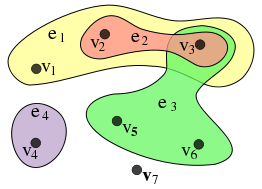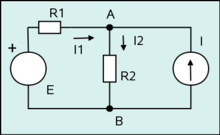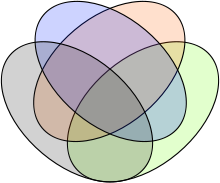# Hypergraph

﻿
HypergraphAn example hypergraph, with X = {v1,v2,v3,v4,v5,v6,v7} and E = {e1,e2,e3,e4} = {{v1,v2,v3}, {v2,v3}, {v3,v5,v6}, {v4}}.

In mathematics, a hypergraph is a generalization of a graph, where an edge can connect any number of vertices. Formally, a hypergraph H is a pair H = (X,E) where X is a set of elements, called nodes or vertices, and E is a set of non-empty subsets of X called hyperedges or links. Therefore, E is a subset of$\mathcal{P}(X) \setminus\{\emptyset\}$, where$\mathcal{P}(X)$ is the power set of X.

While graph edges are pairs of nodes, hyperedges are arbitrary sets of nodes, and can therefore contain an arbitrary number of nodes. However, it is often useful to study hypergraphs where all hyperedges have the same cardinality: a k-uniform hypergraph is a hypergraph such that all its hyperedges have size k. (In other words, it is a collection of sets of size k.) So a 2-uniform hypergraph is a graph, a 3-uniform hypergraph is a collection of triples, and so on.

A hypergraph is also called a set system or a family of sets drawn from the universal set X. Hypergraphs can be viewed as incidence structures and vice versa. In particular, there is a Levi graph corresponding to every hypergraph, and vice versa. In computational geometry, a hypergraph may be called a range space and the hyperedges are called ranges. In cooperative game theory, hypergraphs are called simple games (voting games); this notion is applied to solve problems in social choice theory.

Special cases of hypergraphs include the clutter, where no edge appears as a subset of another edge; and the abstract simplicial complex, which contains all subsets of every edge.

The collection of hypergraphs is a category with hypergraph homomorphisms as morphisms.

## Terminology

Because hypergraph links can have any cardinality, there are multiple, distinct notions of the concept of a subgraph: subhypergraphs, partial hypergraphs and section hypergraphs.

Let H = (X,E) be the hypergraph consisting of vertices$X = \lbrace x_m | m \in M \rbrace$

that is, the vertices are indexed by an index$m\in M$, and the edge set is$E = \lbrace e_i | i\in I, e_i \subseteq X \rbrace$

with the edges ei indexed by an index$i\in I$.

A subhypergraph is a hypergraph with some vertices removed. Formally, the subhypergraph HA induced by a subset A of X is defined as$H_A=\left(A, \lbrace e_i \cap A | e_i \cap A \neq \varnothing \rbrace \right)$

The partial hypergraph is a hypergraph with some edges removed. Given a subset$J \subset I$ of the index set I, the partial hypergraph generated by J is the hypergraph$\left(X, \lbrace e_i | i\in J \rbrace \right)$

Given a subset$A\subseteq X$, the section hypergraph is the partial hypergraph$H \times A = \left(A, \lbrace e_i | i\in I, e_i \subseteq A \rbrace \right)$

The dual H * of H is a hypergraph whose vertices and edges are interchanged, so that the vertices are given by {ei} and whose edges are given by {Xm} where$X_m = \lbrace e_i | x_m \in e_i \rbrace$

When a notion of equality is properly defined, as done below, the operation of taking the dual of a hypergraph is an involution, i.e.,$\left(H^*\right)^* = H$

A connected graph G with the same vertex set as a connected hypergraph H is a host graph for H if every hyperedge of H induces a connected subgraph in G. For a disconnected hypergraph H, G is a host graph if there is a bijection between the connected components of G and of H, such that each connected component G' of G is a host of the corresponding H'.

The primal graph of a hypergraph is the graph with the same vertices of the hypergraph, and edges between all pairs of vertices contained in the same hyperedge. The primal graph is sometimes also known as the Gaifman graph of the hypergraph.

## Bipartite graph model

A hypergraph H may be represented by a bipartite graph BG as follows: the sets X and E are the partitions of BG, and (x1, e1) are connected with an edge if and only if vertex x1 is contained in edge e1 in H. Conversely, any bipartite graph with fixed parts and no unconnected nodes in the second part represents some hypergraph in the manner described above. This bipartite graph is also called incidence graph.

## Isomorphism and equality

A hypergraph homomorphism is a map from the vertex set of one hypergraph to another such that each edge maps to one other edge.

A hypergraph H = (X,E) is isomorphic to a hypergraph G = (Y,F), written as$H \simeq G$ if there exists a bijection$\phi:X \to Y$

and a permutation π of I such that

ϕ(ei) = fπ(i)

The bijection ϕ is then called the isomorphism of the graphs. Note that$H \simeq G$ if and only if$H^* \simeq G^*$.

When the edges of a hypergraph are explicitly labeled, one has the additional notion of strong isomorphism. One says that H is strongly isomorphic to G if the permutation is the identity. One then writes$H \cong G$. Note that all strongly isomorphic graphs are isomorphic, but not vice-versa.

When the vertices of a hypergraph are explicitly labeled, one has the notions of equivalence, and also of equality. One says that H is equivalent to G, and writes$H\equiv G$ if the isomorphism ϕ has

ϕ(xn) = yn

and

ϕ(ei) = fπ(i)

Note that$H\equiv G$ if and only if$H^* \cong G^*$

If, in addition, the permutation π is the identity, one says that H equals G, and writes H = G. Note that, with this definition of equality, graphs are self-dual:$\left(H^*\right) ^* = H$

A hypergraph automorphism is an isomorphism from a vertex set into itself, that is a relabeling of vertices. The set of automorphisms of a hypergraph H (= (XE)) is a group under composition, called the automorphism group of the hypergraph and written Aut(H).

### Examples

Consider the hypergraph H with edges

H = {e1 = {a,b},e2 = {b,c},e3 = {c,d},e4 = {d,a},e5 = {b,d},e6 = {a,c}}

and

G = {f1 = {α,β},f2 = {β,γ},f3 = {γ,δ},f4 = {δ,α},f5 = {α,γ},f6 = {β,δ}}

Then clearly H and G are isomorphic (with ϕ(a) = α, etc.), but they are not strongly isomorphic. So, for example, in H, vertex a meets edges 1, 4 and 6, so that,$e_1 \cap e_4 \cap e_6 = \lbrace a\rbrace$

In graph G, there does not exist any vertex that meets edges 1, 4 and 6:$f_1 \cap f_4 \cap f_6 = \varnothing$

In this example, H and G are equivalent,$H\equiv G$, and the duals are strongly isomorphic:$H^*\cong G^*$.

## Symmetric hypergraphs

The rank r(H) of a hypergraph H is the maximum cardinality of any of the edges in the hypergraph. If all edges have the same cardinality k, the hypergraph is said to be uniform or k-uniform, or is called a k-hypergraph. A graph is just a 2-uniform hypergraph.

The degree d(v) of a vertex v is the number of edges that contain it. H is k-regular if every vertex has degree k.

The dual of a uniform hypergraph is regular and vice-versa.

Two vertices x and y of H are called symmetric if there exists an automorphism such that ϕ(x) = y. Two edges ei and ej are said to be symmetric if there exists an automorphism such that ϕ(ei) = ej.

A hypergraph is said to be vertex-transitive (or vertex-symmetric) if all of its vertices are symmetric. Similarly, a hypergraph is edge-transitive if all edges are symmetric. If a hypergraph is both edge- and vertex-symmetric, then the hypergraph is simply transitive.

Because of hypergraph duality, the study of edge-transitivity is identical to the study of vertex-transitivity.

## Transversals

A transversal or hitting set of a hypergraph H = (X, E) is a set$T\subseteq X$ that has nonempty intersection with every edge. A transversal T is called minimal if no proper subset of T is a transversal. The transversal hypergraph of H is the hypergraph (X, F) whose edge set F consists of all minimal transversals of H. Computing the transversal hypergraph has applications in machine learning and other fields of computer science, as game theory, indexing of databases, SAT problem, data mining and optimization.

## Incidence matrix

Let$V = \{v_1, v_2, ~\ldots, ~ v_n\}$ and$E = \{e_1, e_2, ~ \ldots ~ e_m\}$. Every hypergraph has an$n \times m$ incidence matrix A = (aij) where$a_{ij} = \left\{ \begin{matrix} 1 & \mathrm{if} ~ v_i \in e_j \\ 0 & \mathrm{otherwise}. \end{matrix} \right.$

The transpose At of the incidence matrix defines a hypergraph$H^* = (V^*,\ E^*)$ called the dual of H, where V * is an m-element set and E * is an n-element set of subsets of V * . For$v^*_j \in V^*$ and$e^*_i \in E^*, ~ v^*_j \in e^*_i$ if and only if aij = 1.

## Hypergraph coloring

Hypergraph colouring is defined as follows. Let H = (V,E) be a hypergraph such that$\Vert V\Vert = n$. Then$C=\{c_1, c_2, \ldots, c_n\}$ is a proper colouring of H if and only if, for all$e \in E, \vert e\vert > 1,$ there exists$v_i, v_j \in e$ such that$c_i \neq c_j$.

In other words: For every edge in the graph having at least two nodes as endpoints, the nodes this edge connects are not all of the same color.

## Partitions

A partition theorem due to E. Dauber states that, for an edge-transitive hypergraph H = (X,E), there exists a partition$(X_1, X_2,\cdots,X_K)$

of the vertex set X such that the subhypergraph$H_{X_k}$ generated by Xk is transitive for each$1\le k \le K$, and such that$\sum_{k=1}^K r\left(H_{X_k} \right) = r(H)$

where r(H) is the rank of H.

As a corollary, an edge-transitive hypergraph that is not vertex-transitive is bicolorable.

Graph partitioning (and in particular, hypergraph partitioning) has many applications to IC design and parallel computing.

## Theorems

Many theorems and concepts involving graphs also hold for hypergraphs. Ramsey's theorem and Line graph of a hypergraph are typical examples. Some methods for studying symmetries of graphs extend to hypergraphs.

Two prominent theorems are the Erdős–Ko–Rado theorem and the Kruskal–Katona theorem on uniform hypergraphs.

## Hypergraph drawingThis circuit diagram can be interpreted as a drawing of a hypergraph in which four vertices (depicted as white rectangles and disks) are connected by three hyperedges drawn as trees.

Although hypergraphs are more difficult to draw on paper than graphs, several researchers have studied methods for the visualization of hypergraphs.

In one possible visual representation for hypergraphs, similar to the standard graph drawing style in which curves in the plane are used to depict graph edges, a hypergraph's vertices are depicted as points, disks, or boxes, and its hyperedges are depicted as trees that have the vertices as their leaves. If the vertices are represented as points, the hyperedges may also be shown as smooth curves that connect sets of points, or as simple closed curves that enclose sets of points.An order-4 Venn diagram, which can be interpreted as a subdivision drawing of a hypergraph with 15 vertices (the 15 colored regions) and 4 hyperedges (the 4 ellipses).

In another style of hypergraph visualization, the subdivision model of hypergraph drawing, the plane is subdivided into regions, each of which represents a single vertex of the hypergraph. The hyperedges of the hypergraph are represented by contiguous subsets of these regions, which may be indicated by coloring, by drawing outlines around them, or both. An order-n Venn diagram, for instance, may be viewed as a subdivision drawing of a hypergraph with n hyperedges (the curves defining the diagram) and 2n − 1 vertices (represented by the regions into which these curves subdivide the plane). In contrast with the polynomial-time recognition of planar graphs, it is NP-complete to determine whether a hypergraph has a planar subdivision drawing, but the existence of a drawing of this type may be tested efficiently when the adjacency pattern of the regions is constrained to be a path, cycle, or tree.

## Generalizations

One possible generalization of a hypergraph is to allow edges to point at other edges. There are two variations of this generalization. In one, the edges consist not only of a set of vertices, but may also contain subsets of vertices, ad infinitum. Set membership then provides an ordering, but the ordering is neither a partial order nor a preorder, since it is not transitive. The graph corresponding to the Levi graph of this generalization is a directed acyclic graph. Consider, for example, the generalized hypergraph whose vertex set is V = {a,b} and whose edges are e1 = {a,b} and e2 = {a,e1}. Then, although$b\in e_1$ and$e_1\in e_2$, it is not true that$b\in e_2$. However, the transitive closure of set membership for such hypergraphs does induce a partial order, and "flattens" the hypergraph into a partially ordered set.

Alternately, edges can be allowed to point at other edges, (irrespective of the requirement that the edges be ordered as directed, acyclic graphs). This allows graphs with edge-loops, which need not contain vertices at all. For example, consider the generalized hypergraph consisting of two edges e1 and e2, and zero vertices, so that e1 = {e2} and e2 = {e1}. As this loop is infinitely recursive, sets that are the edges violate the axiom of foundation. In particular, there is no transitive closure of set membership for such hypergraphs. Although such structures may seem strange at first, they can be readily understood by noting that the equivalent generalization of their Levi graph is no longer bipartite, but is rather just some general directed graph.

The generalized incidence matrix for such hypergraphs is, by definition, a square matrix, of a rank equal to the total number of vertices plus edges. Thus, for the above example, the incidence matrix is simply$\left[ \begin{matrix} 0 & 1 \\ 1 & 0 \end{matrix} \right].$

Wikimedia Foundation. 2010.

### Look at other dictionaries:

• Hypergraph — Dieses Stichwortverzeichnis enthält kurze Definitionen und Erklärungen zu den wichtigsten graphentheoretischen Begriffen. A Abstand Siehe: Distanz. Achromatische Zahl Die achromatische Zahl ψ(G) eines Graphen G ist die größte Zahl k, für die G… …   Deutsch Wikipedia

• hypergraph — noun A generalization of a graph, in which edges can connect any number of vertices …   Wiktionary

• Hypergraph-Teleskop — Ein Hypergraph Teleskop ist ein Spiegelteleskop, das ähnlich aufgebaut ist wie ein Cassegrain Teleskop (parabolischer Primärspiegel, hyperbolischer Sekundärspiegel). Um die optische Qualität für die Fotografie zu erhöhen, wird beim Hypergraph wie …   Deutsch Wikipedia

• Line graph of a hypergraph — The line graph of a hypergraph is the graph whose vertex set is the set of the hyperedges of the hypergraph, with two edges adjacent when they have nonempty intersection. In other words, the line graph of a hypergraph is the intersection graph of …   Wikipedia

• Symmetric hypergraph theorem — The Symmetric hypergraph theorem is a theorem in combinatorics that puts an upper bound on the chromatic number of a graph (or hypergraph in general). The original reference for this paper is unknown at the moment, and has been called folklore [R …   Wikipedia

• K-uniformer Hypergraph — Dieser Artikel oder Abschnitt bedarf einer Überarbeitung. Näheres ist auf der Diskussionsseite angegeben. Hilf mit, ihn zu verbessern, und entferne anschließend diese Markierung. Nachbarschaft und Grad sind grundlegende Begriffe der… …   Deutsch Wikipedia

• Uniformer Hypergraph — Dieser Artikel oder Abschnitt bedarf einer Überarbeitung. Näheres ist auf der Diskussionsseite angegeben. Hilf mit, ihn zu verbessern, und entferne anschließend diese Markierung. Nachbarschaft und Grad sind grundlegende Begriffe der… …   Deutsch Wikipedia

• Conformal hypergraph — In graph theory, a branch of mathematics, a hypergraph H is conformal if all the maximal cliques of the 2 section of H are edges of H . Here, the 2 section has an edge F if F contains two vertices and is contained in some edge of H , or if F… …   Wikipedia

• Clique complex — “Whitney complex” redirects here. For the Mississippi sports facility, see Davey Whitney Complex. Clique complexes, flag complexes, and conformal hypergraphs are closely related mathematical objects in graph theory and geometric topology that… …   Wikipedia

• Decomposition method (constraint satisfaction) — In constraint satisfaction, a decomposition method translates a constraint satisfaction problem into another constraint satisfaction problem that is binary and acyclic. Decomposition methods work by grouping variables into sets, and solving a… …   Wikipedia

We are using cookies for the best presentation of our site. Continuing to use this site, you agree with this.